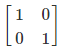# Inverse of a 3 by 3 Matrix

To find the inverse of a 3 by 3 Matrix is a little critical job but can be evaluated by following a few steps. A 3 x 3 matrix has 3 rows and 3 columns. Elements of the matrix are the numbers that make up the matrix. A singular matrix is the one in which the determinant is not equal to zero. For every m×m square matrix there exist an inverse of it. It is represented by M-1. The inverse of a matrix cannot be evaluated by calculators and using shortcuts will be inappropriate. We should practise problems to understand the concept. It has a property as follows:

MM-1 = M-1 M = I2

In the above property, I2 represents the m x m matrix. Suppose, take an example of a 2 x 2 matrix.Any m x m square matrix M, which has zero determinant always has an inverse M-1. It is mostly true for all the square matrix and is given by MM-1 = M-1M =Im

## How to Find the Inverse of 3 x 3 Matrix?

The steps to find the inverse of 3 by 3 matrix

• Compute the determinant of the given matrix
• Calculate the determinant of 2×2 minor matrices
• Formulate the matrix of cofactors
• Take the transpose of the cofactor matrix to get the adjugate matrix
• Finally, divide each term of the adjugate matrix by the determinant

## Inverse Matrix Formula

First, find the determinant of 3 × 3 matrices and then find its minor, cofactors and adjoint and insert the results in the Inverse Matrix formula given below:

$$A^{-1}=\frac{1}{|A|}Adj(A)$$

Where |A| ≠ 0

## Inverse of a 3 x 3 Matrix Example

Let’s see how 3 x 3 matrix looks :

M = $$\begin{bmatrix} a & b &c \\ d& e &f \\ g& h &i \end{bmatrix}$$

Consider the given 3×3 matrix:

$$A =\begin{bmatrix} 1 & 2 &3 \\ 0 & 1 & 4\\ 5 & 6 & 0 \end{bmatrix}$$

Let’s see what are the steps to find Inverse.

### Check the Given Matrix is Invertible

This can be proved if its determinant is non zero. If the determinant of the given matrix is zero, then there is no inverse for the given matrix

det (A) = 1(0-24) -2(0-20) + 3(0-5)

det(A) = -24 +40-15

det (A) = 1

Thus, we can say that the given matrix has an inverse matrix.

### Finding the Determinants of the 2×2 Minor Matrices

Now, we have to find the determinants of each and every 2×2 minor matrices

For first row elements:

$$\begin{bmatrix} 1 & 4\\ 6& 0 \end{bmatrix} = -24$$

$$\begin{bmatrix} 0 & 4\\ 5 & 0 \end{bmatrix} = -20$$

$$\begin{bmatrix} 0 & 1\\ 5 & 6 \end{bmatrix} = -5$$

For second-row elements:

$$\begin{bmatrix} 2 & 3\\ 6 & 0 \end{bmatrix} =-18$$

$$\begin{bmatrix} 1 & 3\\ 5 & 0 \end{bmatrix} =-15$$

$$\begin{bmatrix} 1 & 2\\ 5 & 6 \end{bmatrix} = -4$$

For third-row elements:

$$\begin{bmatrix} 2 & 3\\ 1 & 4 \end{bmatrix} = 5$$

$$\begin{bmatrix} 1 & 3\\ 0 & 4 \end{bmatrix} = 4$$

$$\begin{bmatrix} 1 & 2\\ 0 & 1 \end{bmatrix} = 1$$

Now, the new matrix formed is:

$$A = \begin{bmatrix} -24&-20 &-5 \\ -18& -15 &-4 \\ 5 & 4 & 1 \end{bmatrix}$$

### Formulating the Matrix of Cofactors

Now, to create the adjoint or the adjugated matrix, reverse the sign of the alternating terms as shown below:

The obtained matrix is $$A = \begin{bmatrix} -24&-20 &-5 \\ -18& -15 &-4 \\ 5 & 4 & 1 \end{bmatrix}$$

= $$\begin{bmatrix} -24&-20 &-5 \\ -18& -15 &-4 \\ 5 & 4 & 1 \end{bmatrix}\times \begin{bmatrix}+ &- &+ \\ -& + & -\\ +&- & + \end{bmatrix}$$

=$$\begin{bmatrix} -24&20 &-5 \\ 18& -15 &4 \\ 5 & -4 & 1 \end{bmatrix}$$

### Take the Transpose of the Cofactor Matrix to get the Adjugate Matrix

Now take the transpose of the obtained cofactor matrix.

Thus, $$Adj (A) =\begin{bmatrix} -24 & 18 &5 \\ 20 & -15 & -4\\ -5 & 4 & 1 \end{bmatrix}$$

### Finding the Inverse of the 3×3 Matrix

Now, substitute the value of det (A) and the adj (A) in the formula:

A-1 = (1/1)$$\begin{bmatrix} -24&18 &5 \\ 20& -15 &-4 \\ -5 & 4 & 1 \end{bmatrix}$$
A-1 = (1/1)$$\begin{bmatrix} -24&18 &5 \\ 20& -15 &-4 \\ -5 & 4 & 1 \end{bmatrix}$$